# Sequence

A sequence, list, or stream is a fundamental concept in mathematics and computer science. It is an entity that contains at most countably many other entities, ordered and regarded as a whole.

## Definitions

Precisely, we may wish to define a sequence as an ordered collection of objects from a set$S$, not necessarily distinct, indexed by natural numbers beginning from one. We may also choose to represent a sequence as a function whose domain is either the subset of natural numbers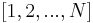$[1,2,...,N]$, or the entire set$\mathbb{N}$. The restriction that the objects be indexed by natural numbers is somewhat artificial; the requirement is really that the elements can, informally be speaking, "lined up", one after another, so that by starting somewhere and going to the next element and the next and so on, any element can eventually be reached. Thus, while sequences may be infinite, they may not be uncountably infinite, so there is no sequence that contains all the real numbers, because the real numbers are uncountable. (Mathematically speaking, a sequence can be assigned an ordinal number from the set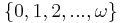$\{0, 1, 2, ..., \omega\}$.) For this reason we may just as well choose to index a sequence by integers starting from zero when it is convenient to do so. The requirement that the elements belong to the same set$S$ is somewhat artificial in mathematics, where we can just take$S$ so that it contains all elements of our sequence anyway. But it is useful in statically typed programming languages to take$S$ to be the set of all objects of a particular type, so that sequences can be uniformly processed using functions that expect or produce a certain type.

The set of sequences of length$n$ over the set$S$ is denoted by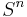$S^n$. The set of infinite sequences taken from the set$S$ may be denoted as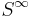$S^{\infty}$,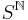$S^{\mathbb{N}}$, or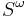$S^{\omega}$. We shall use the notation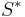$S^*$ for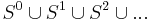$S^0 \cup S^1 \cup S^2 \cup ...$, the set of all finite sequences over the set$S$.

If the set from which the elements of a sequence are taken is finite, and the sequence itself is finite, the sequence is also a string. Sequences, however, can also be infinite and have infinitely many different values for their elements; an example is the Fibonacci sequence.

While in theory, then, a sequence, list, or stream is merely countably many individual values regarded as a whole, the terms list and stream often carry the connotations that they do not support random access efficiently, or perhaps at all. Hence, in certain programming languages, a sequence allowing efficient random access is stored and referred to as an array, a sequence supporting only sequential access is stored as a linked list but often simply referred to as a list, and a (possibly infinite) sequence in which not all the elements might be available right away, such as the sequence of bytes representing standard input, typed on the terminal, is considered a stream. (In a lazy language, such as Haskell, the distinction between the latter two is lost.) We shall assume that the data structure used to represent a sequence is the most appropriate one for the job whenever we discuss algorithms involving sequences.

We shall usually write sequences as their terms in order, separated by commas, and enclosed by square brackets, e.g.,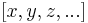$[x, y, z, ...]$ where$x$ is the first element,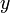$y$ is the second, and so on. The empty sequence is denoted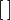$[\,]$.

A sequence over a totally ordered set is said to be increasing if no element is less than the element preceding it (if any). If every element is greater than the element preceding it (unless no such element exists), then the sequence is said to be strictly increasing. Some authors argue that the term increasing is misleading because sequences such as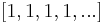$[1,1,1,1,...]$ are considered increasing under this definition, and prefer nondecreasing (because the sequence never decreases). However, all authors agree that the terms decreasing and strictly decreasing have meanings opposite to increasing and strictly increasing, respectively, which leads some authors to counter that the terminology nondecreasing is misleading because the suggested meaning is any sequence that is not decreasing (such as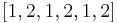$[1,2,1,2,1,2]$, which is neither an increasing nor a decreasing sequence). Because there is no consensus in usage the reader is advised to mind the context in which these terms appear.

The terms prefix, suffix, and concatenation, while strictly applied to strings, may also be defined and used analogously for sequences, simply for lack of better terms. Note that any suffix of an infinite sequence is infinite. Also, the concatenation of an infinite sequence with a nonempty second sequence is undefined, because if it were possible to perform this concatenation, then the first element of the second sequence would have no predecessor in the resulting sequence, which is not allowed. (Mathematically, ordinal numbers greater than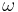$\omega$ are disallowed.)

The term subsequence is not however defined analogously to substring. A subsequence is a sequence obtained by removing some (possibly none, possibly all, possibly infinitely many) terms from a sequence without permuting the remaining terms. Formally, given a sequence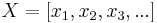$X = [x_1, x_2, x_3, ...]$, to any strictly increasing sequence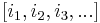$[i_1, i_2, i_3, ...]$ of natural numbers not exceeding the size of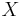$X$, there corresponds a subsequence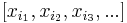$[x_{i_1}, x_{i_2}, x_{i_3}, ...]$ of$X$. A subsequence of an infinite sequence may be either finite or infinite.